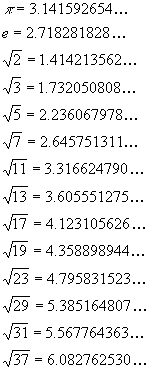# Algebra Formulae

### Algebra

#### Factors and expansions: a, b real; n positive integer

(a + b)2 = a2 + 2ab + b2

(a + b)3 = a3 + 3a2b + 3ab2 + b3

(a + b)4 = a4 + 4a3b + 6a2b2 + 4ab3 + b4

a2 - b2 = (a - b)(a + b)

a2 + b2 = (a - bi)(a + bi)

a3 - b3 = (a - b)(a2 + ab + b2)

a3 + b3 = (a + b)(a2 - ab + b2)

a4 + b4 = (a2 + √2ab + b2)(a2 - √2ab + b2)

an - bn = (a - b)(an-1 + an-2b + ... + bn-1), for n even

an + bn = (a + b)(an-1 - an-2b - ... - bn-1), for n odd

Powers and roots: a > 0, b > 0, x, y real

axay = ax+y

ax / ay = ax-y

axbx = (ab)x

ax / bx = (a / b)x

a-x = 1 / ax

Proportion:

If a / b = c / d, then (a ± b) / b = (c ± d) / d, and (a - b) / (a + b) = (c - d) / (c + d)

Common Irrational Numbers (includes the first 12 prime numbers):To find the root of a number which is not prime – do a prime factorization of the number and multiply / exponentiate appropriately.

For example,Recommended Algebra Books

The classic algebra problem book - very light on theory, plenty of problems with full solutions, more problems with answers

A simplified and updated version of the classic Schaum's Outline.   Not as complete as the previous book, but enough for most students